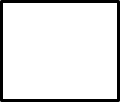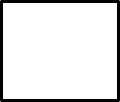1
visibility

Determine the number that makes the equation true.+ 9 = 31 – 6

• A

16

• B

14

• C

17

• D

20

Solution:
Consider the given equation.+ 9 = 31 – 6

To determine the missing number, first, we need to find: 31 – 6.

Which gives:
31 - 6 = 25

Then,+ 9 = 31 – 6+ 9 = 25

Next, to find the missing number we find: 25 – 9.

Which gives:
25 – 9 = 16

So,
16 + 9 = 31 – 6

That is, number 16 makes the equation true.